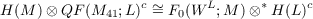#### Vol. 36, No. 1, 1971

 Download this articleFor screen For printingRecent Issues Vol. 325: 1  2 Vol. 324: 1  2 Vol. 323: 1  2 Vol. 322: 1  2 Vol. 321: 1  2 Vol. 320: 1  2 Vol. 319: 1  2 Vol. 318: 1  2Online Archive Volume: Issue:The Journal Subscriptions Editorial Board Officers Contacts Submission Guidelines Submission Form Policies for Authors ISSN: 1945-5844 (e-only) ISSN: 0030-8730 (print) Special Issues Author Index To Appear Other MSP Journals
The Frobenius reciprocity theorem and essentially bounded induced representations

### William Louis Armacost

Vol. 36 (1971), No. 1, 31–43
##### Abstract

Let G be a locally compact group with continuous unitary representations Ri acting on the Hilbert spaces H(Rt),i = 1,2. Suppose that R2 is irreducible. A closed subspace of H(R1), called the null space coming from R1 at R2 and denoted F(R1;R2), is defined. Write c for the conjugate space of the Hilbert space . The following theorem is proved.

Theorem 1. Let G be a compact group with closed subgroup H. Let M,L be irreducible unitary representations of G,H, respectively. Let UL be the induced representation of L, and let ME be the restriction of M to H. Then the following are equivalent:

(a) The classical Frobenius Reciprocity Theorem.

(b) H(M) F(MII;L)cF(UL;M) H(L)c.

When G is not compact, both (a) and (b) may fail. A non-Hilbert Banach space induced representation (WL) is defined. Let G be a locally compact group with closed subgroup H. Let M,L be irreducible unitary representations of G,H, respectively, where H(L) is separable. Spaces F0(WL;M) H(L)c (shown to equal F(UL;M) H(L)c when G is compact) and QF(ME;L) shown to equal F(MH;L) when L is compact) are defined. The following generalization of (b) is shown.

Theorem 2. Let G,H,L be as above. ThenPrimary: 22.60
##### Milestones